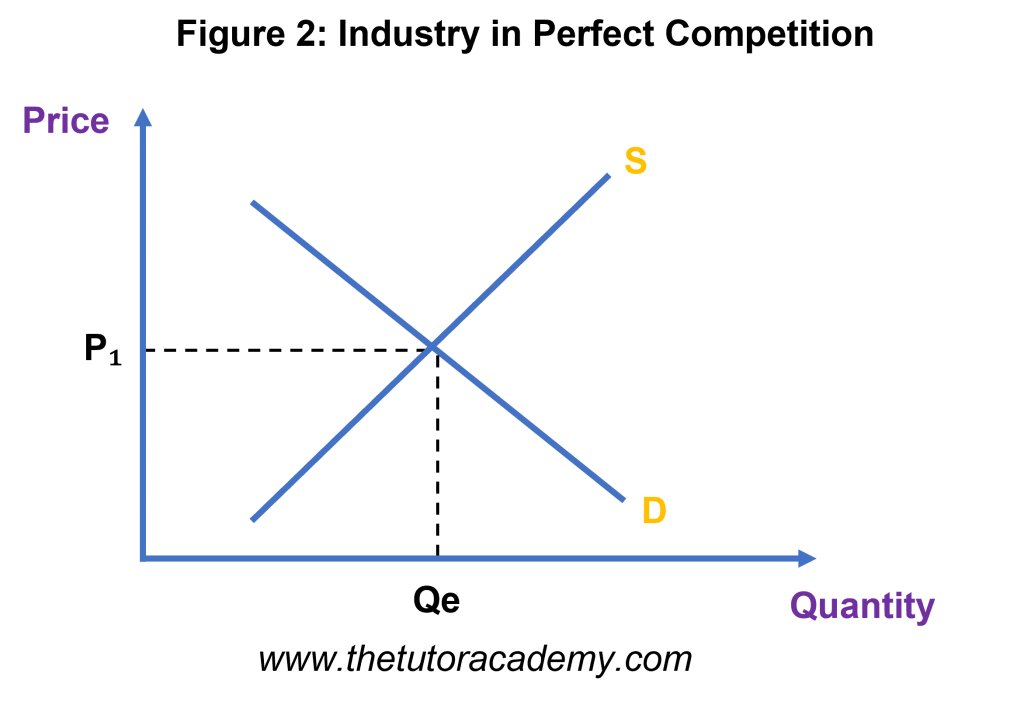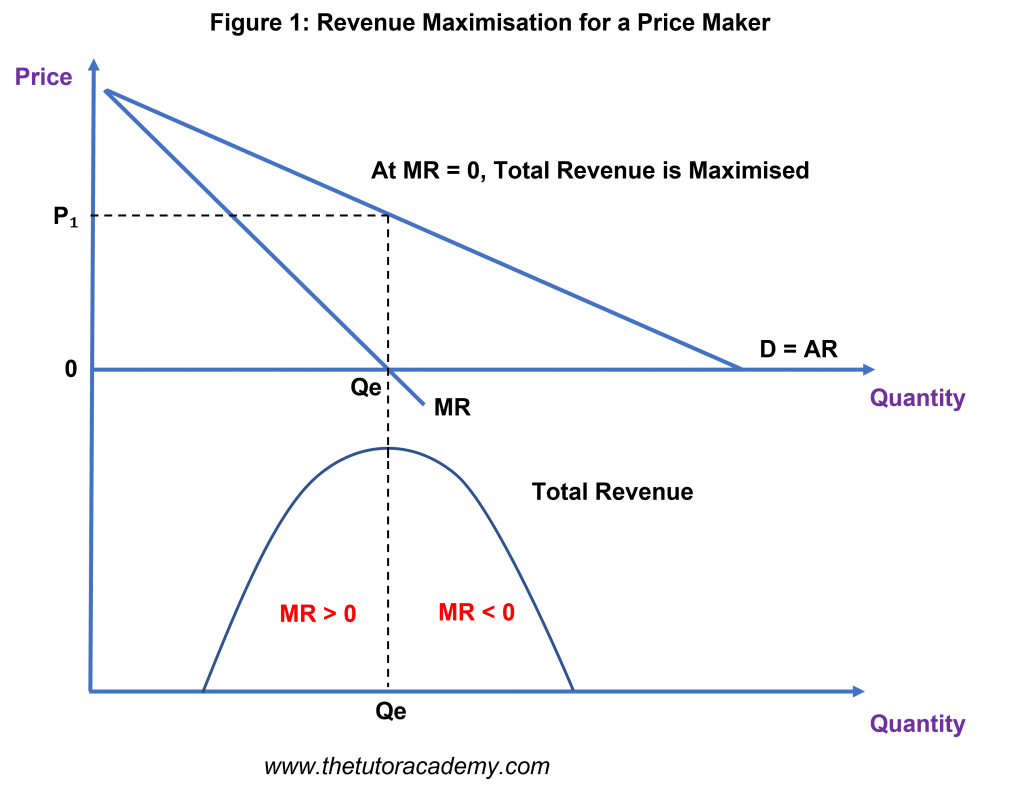# Revenue

Courses Info

Level: AS Levels, A Level, GCSE – Exam Boards: Edexcel, AQA, OCR, WJEC, IB, Eduqas – Economics Revision Notes

### Types of Revenue

#### Total Revenue

The amount of money generated from selling a certain amount of output and is calculated by: price x quantity sold

#### Average Revenue

Revenue per unit and is calculated by: total revenue / quantity sold. Also referred to as the ‘demand’ for a good / service.

#### Marginal Revenue

The revenue generated from selling one extra unit of good / service and can also be known as the difference between total revenue at different levels of output

### Revenue Formula

Revenue = Price x Quantity

### Revenue in a perfectly competitive market (Price Taker)• A firm operating in a competitive market has to set the same price as everyone else in the market
• Price Takers have less power in the market and operate at AR = MR, making normal profits
• Once total revenue has been maximised, it starts to fall with a reduction in prices
• The AR = MR line remains fixed horizontally on the diagram as the demand for a price taker firm is perfectly elastic

### Revenue in a less competitive market – e.g Monopolistic Competition, Oligopoly, Monopoly (Price Maker)• Firms in a less competitive market are able to exercise pricing power and influence the market price
• The total revenue curve for a price maker possesses a ‘parabola’ shape, suggesting that as price falls, the revenue will rise – up to the point of maximum revenue until MR = 0
• A price maker has a downward sloping demand curve and the marginal revenue curve is drawn on the same diagram with a slope twice as steep

## AQA Spec – Additional Content

### Why is the Average Revenue Curve the firm’s demand curve

Average Revenue is the price of a good and therefore also the firm’s demand curve

### The relationship between Marginal Revenue and Total Revenue

Marginal Revenue is calculated by: the change in total revenue / the change in quantity sold and considers the change in quantity sold of goods / services to measure the change in total revenue

Quick Fire Quiz – Knowledge Check

1. Define ‘Total Revenue’ (2 marks)

2. Define ‘Average Revenue’ (2 marks)

3. Define ‘Marginal Revenue’ (2 marks)

4. Draw a revenue diagram, explain what a Price Taker is (6 marks)

5. Draw a revenue diagram, explain what a Price Maker (6 marks)

6. Explain why AR = MR for a Price Taker (3 marks)

7. Explain why the demand curve for a Price Maker is downward sloping (3 marks)

8. Explain the relationship between Total Revenue and the Price Elasticity of Demand (6 marks)

Next Revision Topics

A Level Economics Past PapersEconomics Tutor